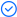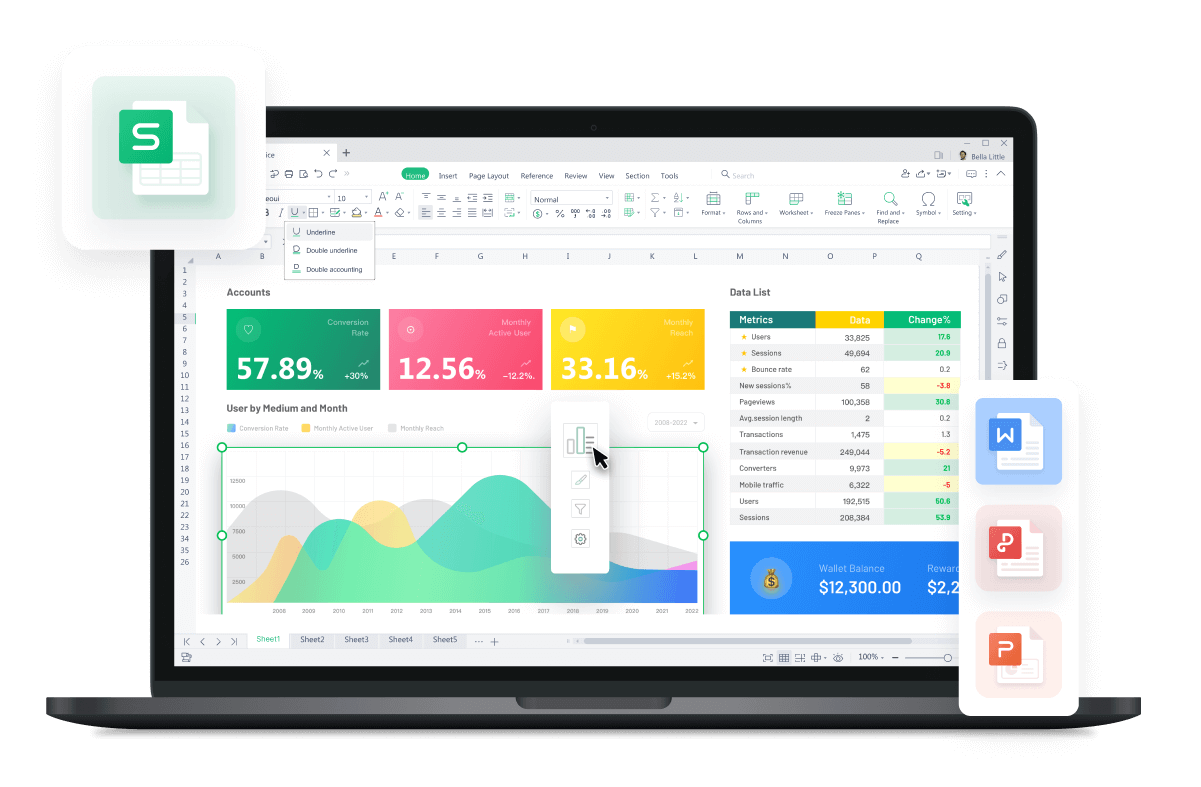WPS Office

Free All-in-One Office Suite with PDF EditorRead, edit, and convert PDFs with the powerful PDF toolkit.Microsoft-like interface, easy to use.

Windows • MacOS • Linux • iOS • Android# How to use left function in excel

August 3, 2022
2.7K Views

If you are wondering how to use left function in excel, it is very easy. But first you should know what does the left function do. The left function primary objective is to extract the information from the LEFT and copy that in the desired cell. That’s it.

Here you will know how to use left function in excel in 3 methods. The working example though will be same but accessing option for the left function will be different.

## 1. Use Left Function Directly by Keyboard Shortcut

1. Open the file you wish to use left function. This example here contains a phone number list with names and contact details. And it is required to extract only the area code.

2. Click on the cell E6 to apply left function.

3. type “=left”

4. Press Tab, the left function is active.

5. Click on the cell D6 for the text.

6. Insert comma and for the number of characters, also include parathesis so “(814)” makes 5 characters. Type 5.

7. press Enter key. The left function has extracted the only the area code.

8. Click at the corner of cell E6, the cursor changes to plus sign.

9. Hold the left mouse button and drag down upto E13 and releas the button. The left function is applied to all contacts.

## 2. Use Left Function Directly by Options

1. Open the file you wish to use left function. This example here contains a phone number list with names and contact details.

2. Click on the cell E6 to apply left function.

3. Click on the Formula tab. This opens the formula ribbon tab.

4. Click on the Text option. A drop down box appears.

5. Click the Left option.

6. A dialogue box appears.

7. Click arrow in the Text field.

8. Click on the cell D6 and hit Enter key. The Text field is assigned value.

9. Again for the number of characters, type 5.

10. Hit Ok.

11. The left function has extracted the only the area code.

12. Click at the corner of cell E6, the cursor changes to plus sign.

13. Hold the left mouse button and drag down upto E13 and release the button. The left function is applied to all contacts.

## 3. Use Left Function Directly by Function Dialogue Box

1. Open the file you wish to use left function. This example here contains a phone number list with names and contact details.

2. Click on the cell E6 to apply left function.

3. Click on the function option.

4. This opens up a dialogue box.

5. In the Search field, type Left. Notice that the left function appears in the search box.

6. Double click it, the left function dialogue box appears.

7. Repeat the same process as before, the left function extracts the area code from the given value.

8. Click at the corner of cell E6, the cursor changes to plus sign.

9. Hold the left mouse button and drag down upto E13 and releas the button. The left function is applied to all contacts.

## Take A Closer Look at Excel LEFT Function

The left function in Excel, which most people don’t know, is useful. Today, we’ll explore what it is with some easy-to-understand examples.

### What is the LEFT function with examples in Excel?

The Excel LEFT function is basically a function that lets people extract a certain number of characters beginning at the left side of a text string.

The function simply allows people to return a certain number of characters (substring) from the start of a string. The formula used for the LEFT function is =LEFT(text, num_chars).

The text is the text string, and the num_chars refers to the number of characters. If the number of characters is omitted, it defaults to 1, resulting in only 1 character being left. If the number of characters is greater than the total length of the text, then it will result in all of the text being left.

Here are perfect examples of Excel left function.

Example 1: If the text string has “She sells seashells by the seashore” as text in the A2 cell, and you use the above-mentioned formula as =LEFT(A2, 3), then the first three characters of text, I.e., “She” will be the result of the LEFT function.

Example 2: If cell B2 has text as “John works harder than Alice” and you use the above-mentioned formula as =LEFT(B2, 4), then the first four characters of text, I.e., “John” will be the results of the LEFT function.

### What is LEFT and Right in Excel?

The LEFT function extracts text from the left side of a text string. Similarly, the right function allows people to extract text from the right side of a text string.

### What is LEFT function used for?

The purpose and use of the LEFT function is to extract a specific number of characters from a long text. People use the Excel LEFT function to make their work more efficient. Imagine you need to extract a few characters of each word or number and that the numbers list is uncountable. What do you do next? The LEFT function can save you worthy time, which you can spend on other productive things.

## Common Mistakes with the LEFT Function and How to Avoid Them

Until now, we have learned the Excel LEFT function, its uses, and its purpose with examples and methods with clear steps on using the left function in Excel. Now it’s time to know what are some common mistakes people make when trying to use the left function in Excel and how you can avoid those mistakes. Here are the mistakes:

### 1. Forgetting to specify the number of characters.

Imagine you have identified and selected the suitable cell and written half the formula correctly, but you forgot to specify the number of characters. What happens when you press the Enter key? Well, the Excel system will use its default which is 1. It means if you wanted to extract three characters and failed to specify the number of characters, it’ll only show one.

For example, if you want to extract “128” from “128-John,” then forgetting to specify the number of characters will result in extracting only 1 number, I.e., “1”.

This can create chaos as it’ll waste your time and energy as now you’ll need to erase them all and follow all the steps again to use the left function correctly. To avoid this, please specify the number of characters you want to extract.

### 2. Using the wrong starting point

You must use the correct starting point to use the left function correctly. Using the correct starting point is essential to avoid any errors and problems. Simply use the correct starting point to avoid any mistakes. Failure to use the correct starting point may waste your time and energy, which could be used on other productive things.

## FAQs about the LEFT Function

### What is the right function in Excel?

Just as there is the left function in Excel, their right function is too. The right function in Excel aims at extracting characters from the texts starting from the right side of the texts. For example, if the text is “567-002” then the right function can be used to extract “002” characters only.

### Are there some examples of the right function?

Yes, there are plenty of examples on the right function. Here are some:

Example 1: If the text is “887-653,” then the right function can be used to extract “653”.

Example 2: Assuming the text is “John-849,” then with the help of the right function, we can extract the characters, I.e., “849”.

### How to use the right function in Excel?

To use the right function in Excel, you need to follow the formula mentioned above. However, you’ll need to replace LEFT with Right. Your formula should look like “=right(text, num_chars) or =right(A2,3)”.

## Summary

The left function basically allows people to extract characters from texts from the left side of the texts. The purpose of the left function is to make work easier and more efficient. Moreover, the function saves time and improves productivity.

Like Excel, WPS Office offers a wide range of functions that allow people to be productive and creative and work efficiently. WPS Office is used by millions of people worldwide. The software offers a user-friendly and easy-to-use interface. Additionally, you can use Word, PPT, Excel, and PDF in the same application. Sounds magical? It is! Download yours now!